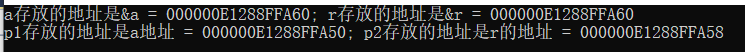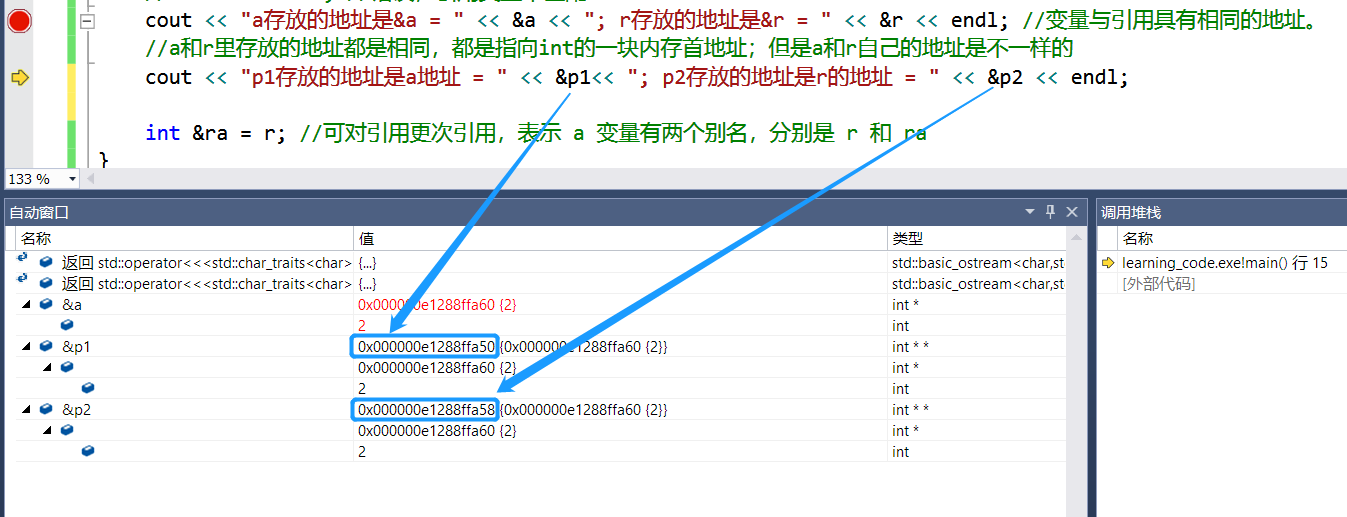## C++中的引用

#include <stdio.h>
#include <iostream>
#include <ctime>
using namespace std;
int main()
{
int a = 2, b;  //变量
int &r = a;  //引用
int *p1 = &a;
int *p2 = &r;
//int &r = b; //错误，不可更改原有的引用关系
//float &rr = b; //错误，引用类型不匹配
cout << "a存放的地址是&a = " << &a << "; r存放的地址是&r = " << &r << endl; //变量与引用具有相同的地址。
//a和r里存放的地址都是相同，都是指向int的一块内存首地址；但是a和r自己的地址是不一样的
cout << "p1存放的地址是a地址 = " << &p1<< "; p2存放的地址是r的地址 = " << &p2 << endl;

int &ra = r; //可对引用更次引用，表示 a 变量有两个别名，分别是 r 和 ra
}### 下面是代码调试过程中的具体细节posted on 2019-10-22 10:05  星辰之衍  阅读(64)  评论(0编辑  收藏